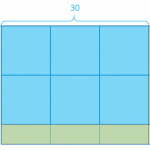Smartick is a fun way to learn math!# I want to learn about: Multiplication and Division

In this section, you will find content about multiplication and division specifically for children in primary school. The best posts about multiplication and division!

Apr13

## What is Multiplication: Steps to Learn and Understand Multiplication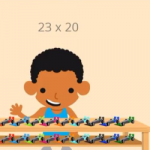What is multiplication? We will explain it in this post! Then we will show some examples using the steps that you should follow to complete multiplication problems, along with a video. What is Multiplication? Multiplication is a mathematical operation that is performed to calculate the result by adding the number as many times as indicated […]

May18

## Learn Everything About the Properties of Powers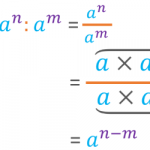In this post, we are going to explain the properties of powers. We will also try to justify the reasoning for following them. Before we begin, let’s remind ourselves of what a power is. What Is a Power? A power is the product of a number multiplied by itself a certain number of times. In […]

Apr06

## The Reason for Divisibility CriteriaSometimes we may be interested in knowing if one number is divisible by another, without having to perform a division. This is especially useful when we work with large numbers that require a lot of time for completing calculations. If you would like to refresh your memory on divisibility criteria, take a look at this […]

Feb03

## Working on Combined Operations through Word ProblemsIn today’s post, we are going to present the way that we work on combined operations through word problems in Smartick. In the field of mathematics in school, combined operations are those problems where we need to use more than one operation. If you don’t remember exactly what they were, click here to refresh your memory! […]

Nov11

## Multiplication of Decimals Using MoneyToday we are going to better understand the multiplication of decimals by using a very common manipulative material: money. It is going to help us understand why multiplying by 10 moves the decimal position to the right. Equivalence of Bills or Coins with Numbers First, we are going to establish the equivalence of each coin […]

Sep09

## Alternative Methods for Multiplication: Russian and Hindu Methods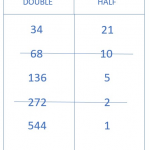In today’s post, we are going to learn two methods to solve multiplication problems that are different from the ones we are used to using. First, we are going to travel to Russia where we will learn how to multiply using the Russian Method. Then, we will continue on to India to learn the Hindu […]

Sep02

## What is Factorial Decomposition?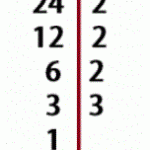In today’s post we are going to answer the question, what is factorial decomposition? I hope that by the end of the post you’ll have it crystal clear. Definition of Factorial Decomposition We are going to start by remembering what a ”factor” is in mathematics. A factor is every number that is multiplied by another. […]

Aug12

## Representing Powers with Rods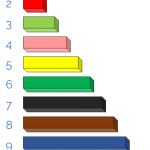In today’s post, I am going to show you how to represent powers with rods. It is fun and easy, you’ll see! We will represent powers to the 2nd and 3rd degree, which are the ones we can reproduce within three dimensions of the physical world. To begin, I will show you a picture of […]

Jul08

## Dividing with Decimals Using Money as an Example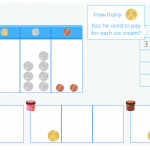In today’s post, you are going to learn how to solve division problems with decimals in the dividend, in other words, dividing a decimal number by a whole number. In order for you to understand the process well, I am going to show you how we explain dividing with decimals in the interactive Smartick tutorial […]

Feb25

## Pedagogical Justification of the Commutative Property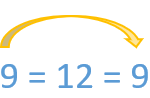In this post, we are going to justify, in a pedagogical sense, the conceptual base of the commutative property and it’s most basic and practical applications. The first thing we need to do is review the concept of the commutative property. For this I have linked some older blog posts about this topic: Applying the […]